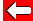# Plotting GraphsWhen plotting graphs, all we need to know is how y depends on x (or x on y). However, there are some simpler graphs that can be drawn where either x or y have just one value.

e.g. y = 2All points along the red line have a y-value of 2. Simple! This means, as you're no doubt aware, that the y-axis is the line x=0 and the x-axis is the line y=0.Go back a pageMaths MenuGo to next page